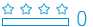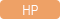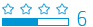cancel
getgamesStudent
1 0 0 0
Message 1 of 4
684
Flag PostHP Recommended

# pv fv calculation wrong answers

hp 10bii+
Microsoft Windows 10 (64-bit)

reset the calculator

shift c 1 p_yr

check sum: 181.181

battery :2.8

version : 3-20-2015 5

N=15

I/YR=9

PMT=90

FV=100

return pv= -752.92

N=15

I/YR=9

PMT=90

FV=1000

return pv= -1000

N=10

I/YR=10

PMT=0

FV=-1000

return pv= 2593.74 (good job!)

N=10

I/YR=6

PMT=1000

PV=-100

return pv= 13001.71(close but no!)

please help me. I wasted so much time on this already.

Tags (2)
3 REPLIES 3Grad Student
190 189 34 64
Message 2 of 4
633
Flag PostHP Recommended

## pv fv calculation wrong answers

Hello,

What answer were you hopping for? Knowing what you are expecting might help understand where things are going wrong.

I also assume that you are trying to calculate FV, not PV?

Doing the calc on other calculators and Excel, I also find -13001.71 (note the - here)...

Cyrille

I am an HP EmployeeGrad Student
124 118 22 45
Message 3 of 4
604
Flag PostHP Recommended

## pv fv calculation wrong answers

As Cyrille has pointed out, it's difficult to know how to help you unless you can give more details about the specific results that don't seem correct to you.

That said, there are some general concepts that are frequently the cause of unexpected results in TVM calculators. In particular, be very careful to understand the application of the following:

1) Sign Convention: generally speaking, amounts being paid or invested (coming out of your funds) are entered as negative values. Values that are returned to you in the form of payments or final returns are generally positive. Interest rates have their own sign convention as well, with negative values implying depreciation vs. appreciation for positive ones. Note that changing the sign of a value on HP calculators usually involves entering the digits first, then pressing a key labeled "+/-" or "CHS" (as opposed to any use of the "-" key).

2) BEG/END Mode: if regular payments are involved, different formulas are used by your calculator depending on the timing of the payments (at the beginning of each period or the end). Make sure that you've got the proper mode set prior to solving for your end result.

3) Interest Rate Assignment: it is critical to understand the proper use and application of interest rate conventions. Most people are already aware that interest rates are generally entered as a percentage (5% is entered as 5 instead of 0.05), but it's common to overlook the importance of periods-per-year, which may either require additional calculations or separate variable entry (depends on which model calculator you use). Somewhat less common, but perhaps even more vexing, is the concept of mis-matched compounding periods for rates and payments (an example of this is Canadian mortgages). These issues are handled differently by different models of calculators, so you should seek out documentation and examples specific to your model if you are attempting to solve that type of problem.

Your original post listed a periods-per-year setting of 1, which we can only assume applies to all of the following examples. I didn't see any specifics about BEG/END mode, however, so any of the examples that have a non-zero payment should be assessed for the appropriate setting when evaluating results. In the following comments, I've listed separate results for the two modes if payments were involved. I've also shown all digits of results so that rounding issues may be more apparent.

Ex. 1: N=15, I/YR=9, PMT=90, FV=100

BEG MODE PV: -818.2073391
END MODE PV: -752.915762818

Your result matches the END mode solution.

Ex. 2: N=15, I/YR=9, PMT=90, FV=1000

BEG MODE PV: -1065.29157628
END MODE PV: -1000

Your result matches the END mode solution.

Ex. 3: N=10, I/YR=10, PMT=0, PV=-1000

FV: 2593.7424601

Since PMT=0, BEG/END mode doesn't apply. Your result of 2593.74 only makes sense if you swap the data for what you've labeled as FV and PV (which is what I did above); I assume this was simply a typo.

Ex. 4: N=10, I/YR=6, PMT=1000, PV=-100

BEG MODE FV: -13792.5578693
END MODE FV: -13001.7101727

I'm assuming this example was meant to calculate the FV instead of the PV. Your result matches the END mode solution. Were you instead expecting the BEG mode result? If so, make sure to set that mode prior to solving for FV.

Hope this helps!

MONSI1Student
1 1 0 0
Message 4 of 4
234
Flag PostHP Recommended

## pv fv calculation wrong answers

I have had the same issue adding my wepage 

† The opinions expressed above are the personal opinions of the authors, not of HP. By using this site, you accept the Terms of Use and Rules of Participation